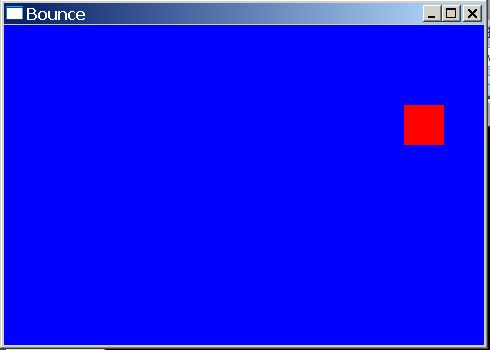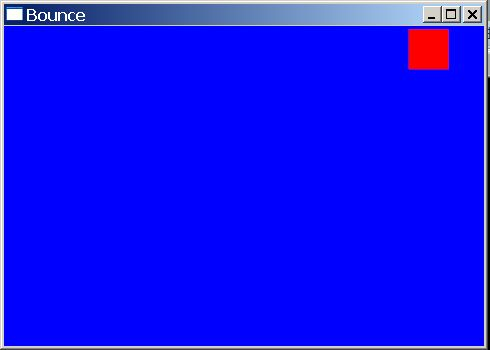# 使用OpenGL和GLUT，结合双缓冲区生成动画

双缓冲区的特性，允许在一个屏幕之外的缓冲区中执行绘图代码，然后使用一条交换命令把完成绘制的图形立即显示在屏幕上。

双缓冲模式的用途：

1、  使用双缓冲，可以合成一幅图像，并在完成之后再显示。用户绝不会看到一幅不完整的图像，因为图像只有在完全完成之后才会在屏幕上显示。

2、  使用双缓冲，可以实现动画效果。每帧的图像都在屏幕之外的缓冲区中绘制，等绘图完成之后快速交换到屏幕中，如此反复，生成动画效果。

/* 程序清单 2-3

*/

#include<glut.h>

// 方块的起始位置和大小

GLfloat x1 = 0.0f;

GLfloat y1 = 0.0f;

GLfloat rsize = 25.0f;

// xy方向的步进大小

GLfloat xstep = 1.0f;

GLfloat ystep = 1.0f;

// 窗口的大小（使用逻辑笛卡尔坐标系统）

GLfloat windowWidth;

GLfloat windowHeight;

// 绘制场景（显示回调函数）

void RenderScene()

{

// OpenGL命令，清除颜色缓冲区（使用当前设置的颜色）

glClear(GL_COLOR_BUFFER_BIT);

// 把当前绘图颜色设置为红色

glColor3f(1.0f, 0.0f, 0.0f);

// OpenGL命令，用当前的绘图颜色绘制一个填充矩形（提供左上角和右下角的顶点坐标）

glRectf(x1, y1, x1 + rsize, y1 - rsize);

// 刷新绘图命令，此时所有未执行的OpenGL命令被执行

glutSwapBuffers();

}

// GLUT函数库调用，计时器函数

void TimerFunction(int value)

{

// 在到达右边或者左边时翻转方向，这里的边界选取要结合裁剪区域的定义

if (x1 >windowWidth - rsize ||

x1 < -windowWidth) {

xstep = -xstep;

}

// 在到达上边或者下边时翻转方向，这里的边界选取要结合裁剪区域的定义

if (y1 >windowHeight ||

y1 < -windowHeight + rsize) {

ystep = -ystep;

}

// 移动方块

x1 += xstep;

y1 += ystep;

// 检查边界，防止方块在反弹时窗口变小，使方块出现在新的裁剪区域之外

if (x1 >(windowWidth - rsize + xstep)) {

x1 = windowWidth - rsize -1;

} elseif (x1 < -windowWidth - xstep) {

x1 = -windowWidth - 1;

}

// 检查边界，防止方块在反弹时窗口变小，使方块出现在新的裁剪区域之外

if (y1 >(windowHeight + ystep)) {

y1 = windowHeight - 1;

} elseif (y1 < -windowHeight + rsize - ystep) {

y1 = -windowHeight + rsize -1;

}

// 使用新的坐标重新绘制场景

glutPostRedisplay();

// 重新设置计时器函数

glutTimerFunc(33, TimerFunction, 1);

}

// 设置渲染状态

void SetupRC()

{

// 设置用于清除窗口的颜色

glClearColor(0.0f, 0.0f, 1.0f, 1.0f);

}

// 当窗口大小改变时由GLUT函数库调用

voidChangeSize(GLsizei w, GLsizei h)

{

// 纵横比

GLfloat aspectRatio;

// 防止被0所除

if (0 == h){

h = 1;

}

// 设置视口为窗口的大小

glViewport(0, 0, w, h);

// 选择投影矩阵，并重置坐标系统

glMatrixMode(GL_PROJECTION);

glLoadIdentity();

// 计算窗口的纵横比（像素比）

aspectRatio = (GLfloat) w / (GLfloat) h;

// 定义裁剪区域（根据窗口的纵横比，并使用正投影）

if (w <=h) {// <

windowWidth = 100.0 ;

windowHeight = 100.0 / aspectRatio;

glOrtho(-100.0, 100.0, -100 /aspectRatio, 100 / aspectRatio, 1.0, -1.0);

} else {// >

windowWidth = 100.0 * aspectRatio;

windowHeight = 100.0;

glOrtho(-100.0 * aspectRatio, 100.0 *aspectRatio, -100.0, 100.0, 1.0, -1.0);

}

// 选择模型视图矩阵，并重置坐标系统

glMatrixMode(GL_MODELVIEW);

glLoadIdentity();

}

int main(int argc,char *argv[])

{

// 传递命令行参数，并对GLUT函数库进行初始化

glutInit(&argc, argv);

// 设置创建窗口时的显示模式（双缓冲区、RGB颜色模式）

glutInitDisplayMode(GLUT_DOUBLE |GLUT_RGB);

// 设置窗口的初始大小

glutInitWindowSize(480, 320);

// 创建窗口

glutCreateWindow("Bounce");

// 设置显示回调函数

glutDisplayFunc(RenderScene);

// 设置当窗口的大小发生变化时的回调函数

glutReshapeFunc(ChangeSize);

// 设置计时器函数

glutTimerFunc(33, TimerFunction, 1);

// 设置渲染状态

SetupRC();

// 启动GLUT框架的运行，一经调用便不再返回，直到程序终止

glutMainLoop();

return 0;

}nice~